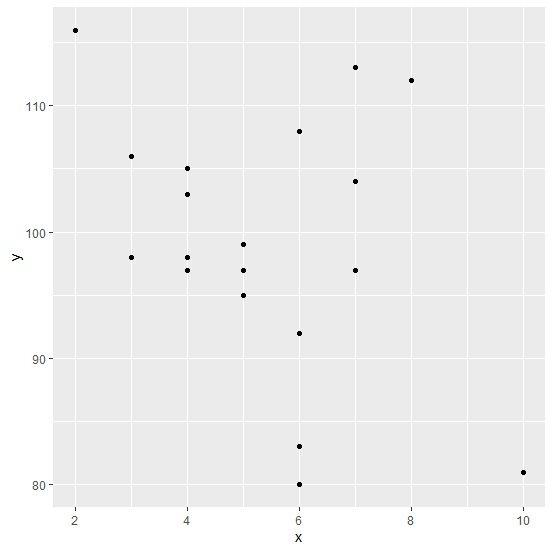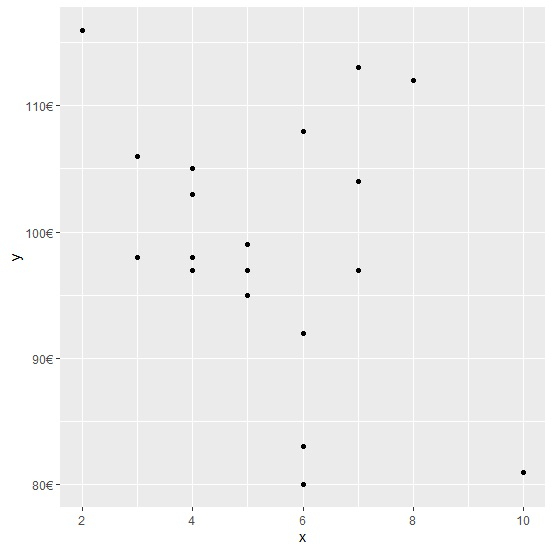# How to display Y-axis with Euro sign using ggplot2 in R?

When we have a Euro currency column in an R data frame as a response variable then we might to display the Euro sign in the plot created by using ggplot2 package. For this purpose, we can use scales package and the scale for Y axis will be changed by using the command scale_y_continuous(labels=dollar_format(suffix="€",prefix="")) to the plot command.

Consider the below data frame −

## Example

Live Demo

x<-rpois(20,5)
y<-rpois(20,100)
df<-data.frame(x,y)
df

## Output

   x   y
1  8   112
2  10  81
3  4   97
4  7   104
5  4   98
6  5   99
7  5   97
8  7   97
9  5   95
10 3   98
11 6   92
12 6   80
13 6   92
14 6  108
15 7  113
16 4  103
17 3  106
18 2  116
19 4  105
20 6  83

Loading ggplot2 package and creating a scatterplot between x and y −

## Example

library(ggplot2)
ggplot(df,aes(x,y))+geom_point()

## OutputLoading scales package and creating the scatterplot with Euro sign displayed with Y-axis labels −

## Example

library(scales)
ggplot(df)+geom_point(aes(x,y))+scale_y_continuous(labels=dollar_format(suffix="€",prefix=""))

## Output